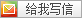# 5652. Snow White and the N dwarfs

Seter posted @ 2011年11月26日 21:45 in SPOJ with tags Dichotomize&Check Classical , 2280 阅读
ID DATE USER PROBLEM RESULT TIME MEM LANG
6087529 2011-11-26 13:51:59 Seter Snow White and the N dwarfs accepted
edit  run
3.89 29M

C

SPOJ上PCOUNT的冉克一被别的神犇夺走了……这么多天终于又有个冉克一了……

PS：BZOJ上这题我Rank2……ymRank1的……

#include <stdio.h>
#include <stdlib.h>
int n,c,cs,a,rs,rk,m;
char str,*p=str;
inline getint(){
int re=0;
while(*p<'0'||*p>'9')p++;
while(*p>='0'&&*p<='9')re=re*10+*p++-48;
return re;
}main(){
for(n=getint(),cs=32-__builtin_clz(c=getint()+1);++i!=n;j=-1)for(m[(rs[i]=t=getint())+1]++;++j!=cs;t>>=1)a[i+1][j]=a[i][j]+(t&1);
for(i=1;i++!=c;m[i]+=m[i-1]);
for(i=-1;++i!=n;rk[m[rs[i]]++]=i)rk[m[rs[i]]]=rs[i];
for(t=getint();t--;){
A=getint()-1;B=getint();j=B-A>>1;ans=0;
for(i=-1;++i!=cs;)if(a[B][i]-a[A][i]<<1==B-A){ans=0;break;}else if(a[B][i]-a[A][i]>j)ans+=1<<i;
if(!ans||ans>=c||m[ans]==m[ans-1]){puts("no");continue;}
for(l=m[ans-1]-1,r=m[ans]-1;l+1!=r;rk[mid]>A?(r=mid):(l=mid))if(rk[mid=1+l+r>>1]==A)break;
k=1+l+r>>1;
for(l=m[ans-1],r=m[ans];l+1!=r;rk[mid]>B-1?(r=mid):(l=mid))if(rk[mid=l+r>>1]==B-1)break;
if((l+r>>1)-k>=j)printf("yes %d\n",ans);else puts("no");
}return 0;
}By Seterxiaodao 说:
2011年11月30日 08:33

Seter 快去怒艹 <a href="http://www.spoj.pl/problems/XXXXXXXX/">XXXXXXXX</a>

Seter 说:
2011年11月30日 12:43(输入验证码)
or Ctrl+Enter
Host by is-Programmer.com | Power by Chito 1.3.3 beta | © 2007 LinuxGem | Design by Matthew "Agent Spork" McGee